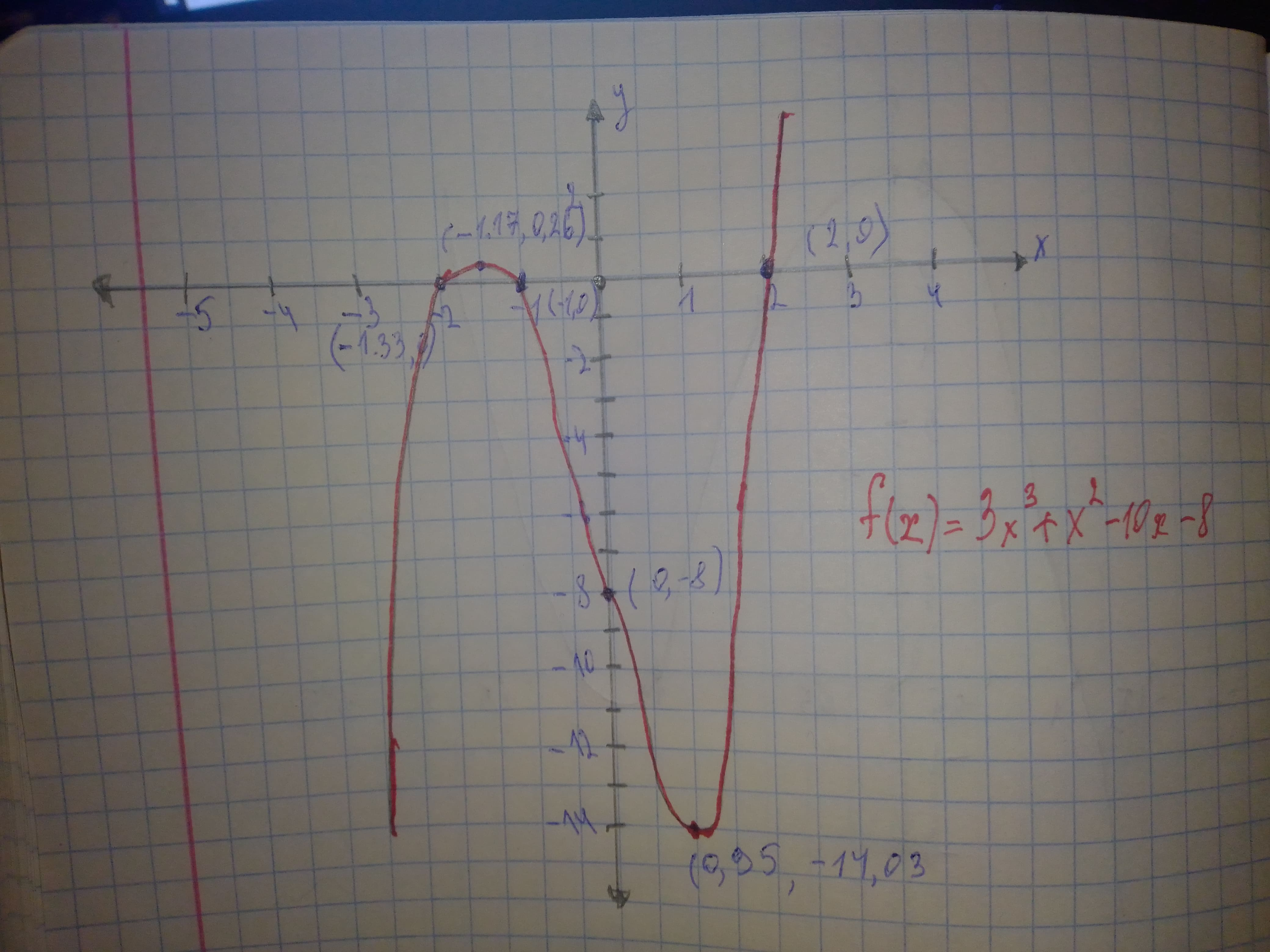# For each polynomial function, one zero is given. Find all rational zeros and factor the polynomial. Then graph the function. f(x)=3x^{3}+x^{2}-10x-8, zero:2illusiia 2020-12-06 Answered

For each polynomial function, one zero is given. Find all rational zeros and factor the polynomial. Then graph the function. $f\left(x\right)=3{x}^{3}+{x}^{2}-10x-8$, zero:2

You can still ask an expert for help

• Questions are typically answered in as fast as 30 minutes

Solve your problem for the price of one coffee

• Math expert for every subject
• Pay only if we can solve itsovienesY

Step 1 Since 2 is a zero of $f\left(x\right)=3{x}^{3}+{x}^{2}-10x-8$ The quotient is $3{x}^{2}+7x+4$
$f\left(x\right)=\left(x-2\right)\left(3{x}^{2}+7x+4\right)$ Factor the trinomial $3{x}^{2}+7x+4=\left(3x+4\right)\left(x+1\right)$ So, the function in factored form is $f\left(x\right)=\left(x-2\right)\left(3x+4\right)\left(x+1\right)$ Set each factor equal to zero $x-2=0$ or $3x+4=0$ or $x+1=0$ So, all rational zeros are $2,-\frac{4}{2},-1$ Step 2 Since, all rational zeros are $2,-\frac{4}{3},-1$ So, the graph of the function crosses the x-axis at $\left(2,0\right),\left(-\frac{4}{3},0\right),\left(-1,0\right)$ To find the y-intercept evaluate f(0) $f\left(x\right)=3{x}^{3}+{x}^{2}-10x-8$
$f\left(0\right)=3{\left(0\right)}^{3}+{\left(0\right)}^{2}-10\left(0\right)-8$ Substitute 0 for x $=-8$ The leading coefficient is 3 (positive), and the function of degree 3 (odd) So, the end behavior is $x\to \mathrm{\infty },f\left(x\right)\to \mathrm{\infty }$ $x\to -\mathrm{\infty },f\left(x\right)\to -\mathrm{\infty }$ See the graph of f(x)